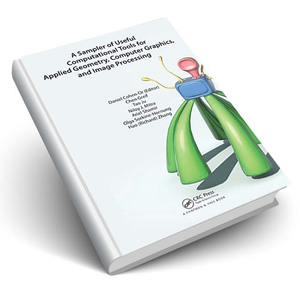# A Sampler of Useful Computational Tools for Applied Geometry, Computer Graphics, and Image Processing

• \$9.99

A Sampler of Useful Computational Tools for Applied Geometry, Computer Graphics, and Image Processing shows how to use a collection of mathematical techniques to solve important problems in applied mathematics and computer science areas. The book discusses fundamental tools in analytical geometry and linear algebra. It covers a wide range of topics, from matrix decomposition to curvature analysis and principal component analysis to dimensionality reduction.